# 机器学习Machine Learning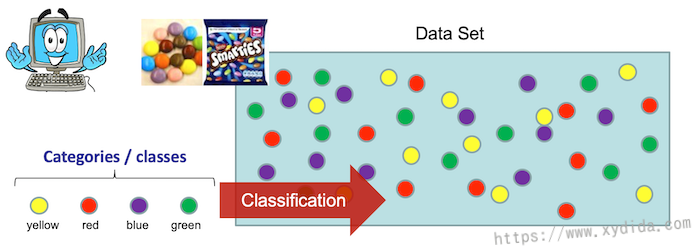## 监督式分类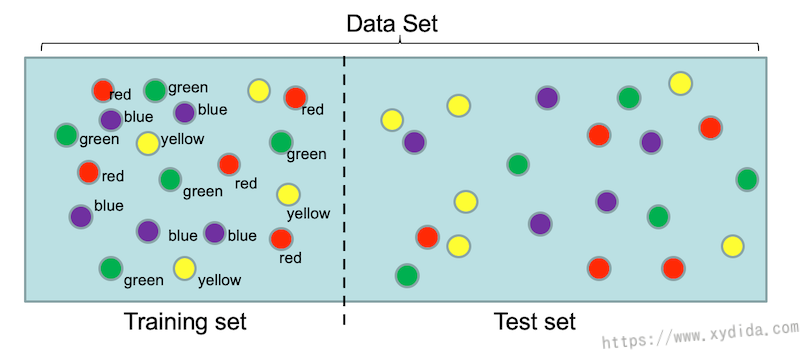## 非监督式分类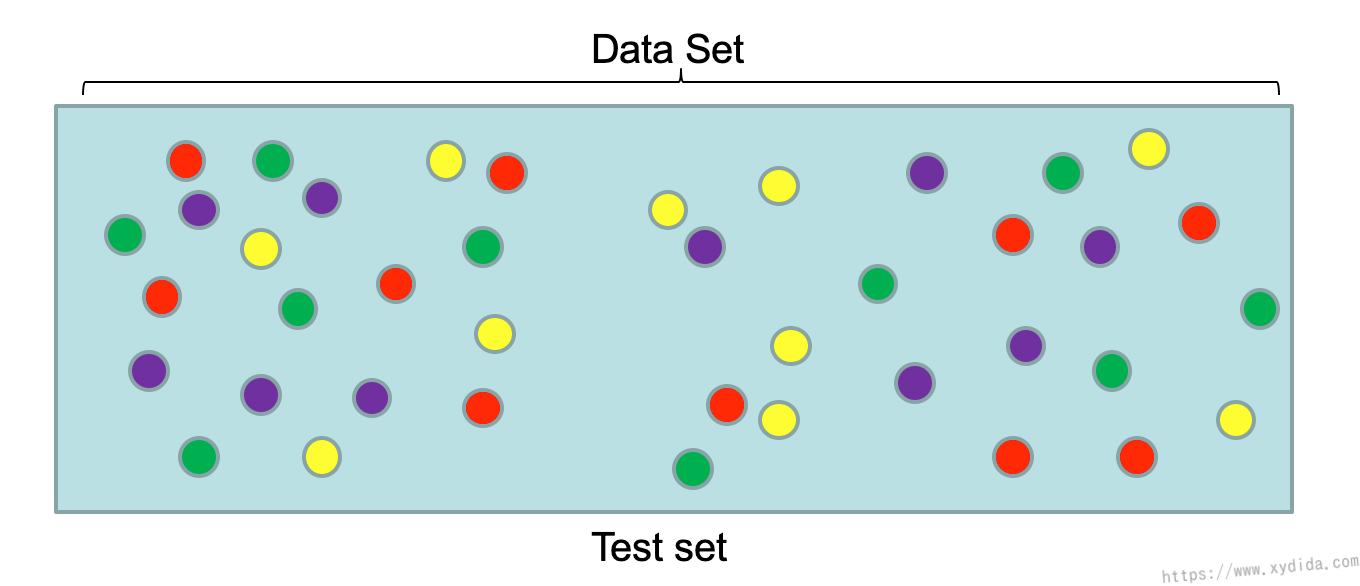# 结果评估 Evaluation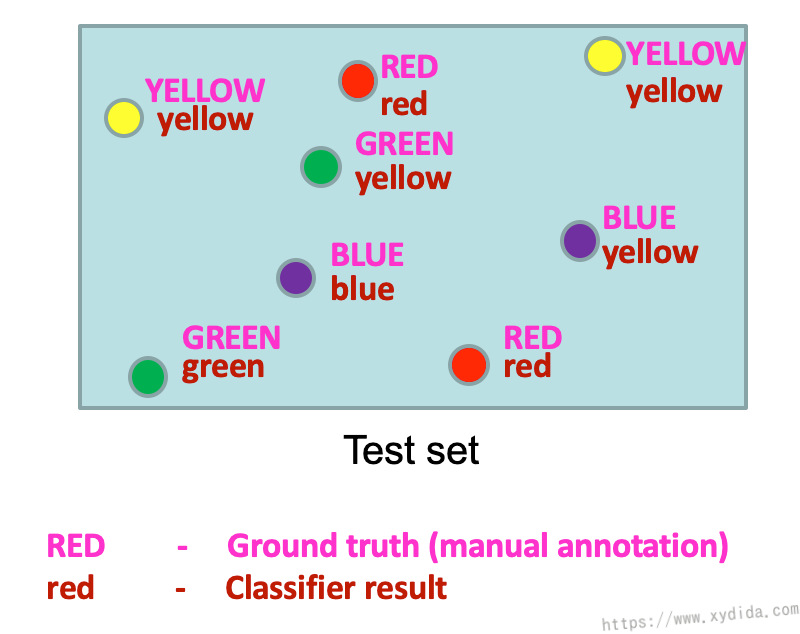## Confusion Matrix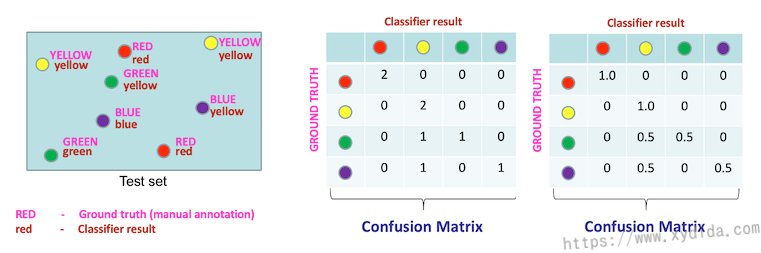Confusion Matrix通常用来统计各个分类预测的频率，可以看出哪些分类机器预测的好，哪些预测的不好。表格中每一行代表真实的分类，每一列代表预测的分类，我们对每一行进行归一化处理，如果预测结果是正确的，归一化后就是1，数值越小，正确预测就越低。上图中绿色和紫色的精确度只有50%，绿色和紫色都容易被识别成黄色。表格中的对角线数值就是该分类下的准确度。对每一个分类的精度求一个平均就是Mean Average Precision (MAP)，MAP代表了整体的精度，比如上图中的MAP值为：

$\frac{1 + 1 + 0.5 + 0.5}{4} = 75\%$

# OverfittingOverfitting是训练中常常会遇到的一个难题，在训练时，往往会追求更高的精确度，这就导致模型学习该训练集下更多的特征，其中就包括了一些无用的特征，因为这些特征只对当前数据有用，换一个新的数据就起不到辨别的作用，总结下来就是模型的通用性（generalization）不强，死记硬背了很多特征。就好比考试，平时做题只背答案，习题集上都是满分，可一考试都不会，因为这些题都没见过呀。Overfitting在测试时的反应就是错误率很高，训练时的错误率很低。

# Underfitting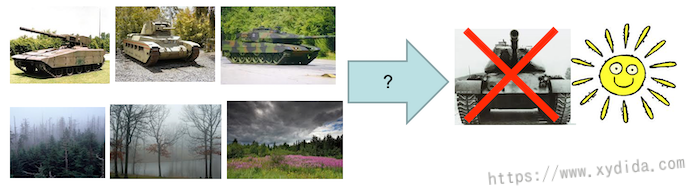# 计算距离

## L2距离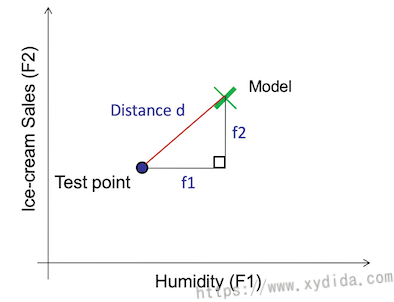L2距离又称欧氏距离，可以解释为连接两个点的线段的长度。在二维空间中，公式如下：

$d = \sqrt{(x_2 - x_1)^2 + (y_2 - y_1)^2}$

$d = \sqrt{(x_2 - x_1)^2 + (y_2 - y_1)^2 + (z_2 - z_1)^2}$

$D(x,y) = \sqrt{\sum_{i=1}^{n}(x_i - y_i)^2}$

L2距离并不是尺度不变的，意味着计算的距离会根据特征的单位发生倾斜，在计算前，通常会将数值归一化。但是，随着数据维数增加，会造成维数灾难（curse of dimension）。

## L1距离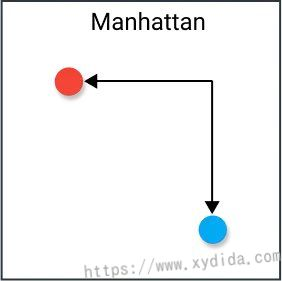L1距离又称曼哈顿距离、出租车距离、城市距离，计算公式如下：

$D(x,y) = \sum_{i=1}^{n}\lvert{x_i - y_i}\rvert$